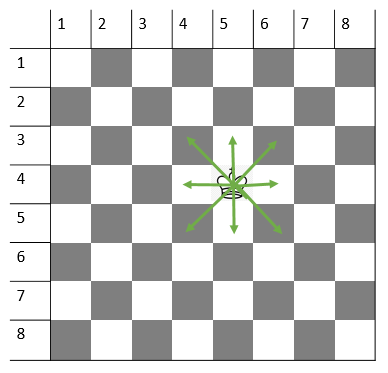Related Articles
Total position where king can reach on a chessboard in exactly M moves | Set 2
• Last Updated : 01 Apr, 2019

Given the position of the king on an 8 X 8 chessboard, the task is to count the total number of squares that can be visited by the king in m moves. The position of the king is denoted using row and column number.
Note: The square which is currently acquired by the king is already visited and will be counted in the result.

Examples:

Input: r = 4, c = 4, m = 1
Output: 9

Input: r = 4, c = 4, m = 2
Output: 25

## Recommended: Please try your approach on {IDE} first, before moving on to the solution.

Approach: A king can move one square in any direction (i.e horizontally, vertically and diagonally). So, in one move king can visit its adjacent squares.So, A square which is within m units distance (Considering 1 Square as 1 unit distance) from the king’s current position can be visited in m moves.

1. For all squares of the chessboard, check if a particular square is at m unit distance away or less from King’s current position.
2. Increment count, if step 1 is true.
3. Print the count

Below is the implementation of the above approach:

## C++

 `// C++ implementation of the approach ` `#include ` `using` `namespace` `std; ` ` `  `// Function to return the count of squares ` `// that can be visited by king in m moves ` `int` `countSquares(``int` `r, ``int` `c, ``int` `m) ` `{ ` ` `  `    ``// To store the count of squares ` `    ``int` `squares = 0; ` ` `  `    ``// Check all squares of ` `    ``// the chessboard ` `    ``for` `(``int` `i = 1; i <= 8; i++) { ` `        ``for` `(``int` `j = 1; j <= 8; j++) { ` ` `  `            ``// Check if square (i, j) is ` `            ``// at a distance <= m units ` `            ``// from king's current position ` `            ``if` `(max(``abs``(i - r), ``abs``(j - c)) <= m) ` `                ``squares++; ` `        ``} ` `    ``} ` ` `  `    ``// Return count of squares ` `    ``return` `squares; ` `} ` ` `  `// Driver code ` `int` `main() ` `{ ` `    ``int` `r = 4, c = 4, m = 1; ` ` `  `    ``cout << countSquares(r, c, m) << endl; ` ` `  `    ``return` `0; ` `} `

## Java

 `// Java implementation of the approach ` `class` `GFG { ` ` `  `    ``// Function to return the count of squares ` `    ``// that can be visited by king in m moves ` `    ``static` `int` `countSquares(``int` `r, ``int` `c, ``int` `m) ` `    ``{ ` `        ``// To store the count of squares ` `        ``int` `squares = ``0``; ` ` `  `        ``// Check all squares of ` `        ``// the chessboard ` `        ``for` `(``int` `i = ``1``; i <= ``8``; i++) { ` `            ``for` `(``int` `j = ``1``; j <= ``8``; j++) { ` ` `  `                ``// Check if square (i, j) is ` `                ``// at a distance <= m units ` `                ``// from king's current position ` `                ``if` `(Math.max(Math.abs(i - r), Math.abs(j - c)) <= m) ` `                    ``squares++; ` `            ``} ` `        ``} ` ` `  `        ``// Return count of squares ` `        ``return` `squares; ` `    ``} ` ` `  `    ``// Driver code ` `    ``public` `static` `void` `main(String[] args) ` `    ``{ ` `        ``int` `r = ``4``, c = ``4``, m = ``1``; ` `        ``System.out.print(countSquares(r, c, m)); ` `    ``} ` `} `

## C#

 `// C# implementation of the approach ` `using` `System; ` `class` `GFG { ` ` `  `    ``// Function to return the count of squares ` `    ``// that can be visited by king in m moves ` `    ``static` `int` `countSquares(``int` `r, ``int` `c, ``int` `m) ` `    ``{ ` `        ``// To store the count of squares ` `        ``int` `squares = 0; ` ` `  `        ``// Check all squares of ` `        ``// the chessboard ` `        ``for` `(``int` `i = 1; i <= 8; i++) { ` `            ``for` `(``int` `j = 1; j <= 8; j++) { ` ` `  `                ``// Check if square (i, j) is ` `                ``// at a distance <= m units ` `                ``// from king's current position ` `                ``if` `(Math.Max(Math.Abs(i - r), Math.Abs(j - c)) <= m) ` `                    ``squares++; ` `            ``} ` `        ``} ` ` `  `        ``// Return count of squares ` `        ``return` `squares; ` `    ``} ` ` `  `    ``// Driver code ` `    ``public` `static` `void` `Main() ` `    ``{ ` `        ``int` `r = 4, c = 4, m = 1; ` `        ``Console.Write(countSquares(r, c, m)); ` `    ``} ` `} `

## Python3

 `# Python implementation of the approach ` ` `  `# Function to return the count of squares ` `# that can be visited by king in m moves ` `def` `countSquares(r, c, m): ` ` `  `    ``# To store the count of squares ` `    ``squares ``=` `0` `     `  `    ``# Check all squares of  ` `    ``# the chessboard ` `    ``for` `i ``in` `range` `(``1``, ``9``): ` `        ``for` `j ``in` `range` `(``1``, ``9``): ` `             `  `            ``# Check if square (i, j) is ` `            ``# at a distance <= m units ` `            ``# from king's current position ` `            ``if``(``max``(``abs``(i ``-` `r), ``abs``(j ``-` `c)) <``=` `m): ` `                ``squares ``=` `squares ``+` `1` `         `  `    ``# Return count of squares ` `    ``return` `squares ` ` `  `# Driver code ` `r ``=` `4` `c ``=` `4` `m ``=` `1` ` `  `print``(countSquares(r, c, m)); `

## PHP

 ` `

Output:

```9
```

Attention reader! Don’t stop learning now. Get hold of all the important DSA concepts with the DSA Self Paced Course at a student-friendly price and become industry ready.

My Personal Notes arrow_drop_up
Recommended Articles
Page :